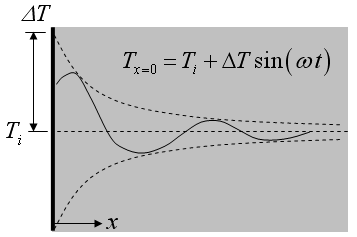Contents - Index

Periodic TemperatureFunction semiinf5(T_i,DeltaT,omega,alpha,x,time) returns the temperature for a given position and time within a semi-infinite body with a sinusoidally varying surface temperature using the relation provided in Table 3-2 of Nellis and Klein.

Inputs:
T_i - initial temperature of the solid  [C] or [K]
DELTAT - amplitude of surface temperature variation [C] or [K]
omega - angular velocity [deg/s] or [rad/s] depending on the unit setting in EES.   The surface temperature is T_i+DELTAT*sin(omega*time)
alpha - thermal diffusivity (m^2/s)
x - perpendicular distance from surface [m]
time - time in  [s]

Note that this function can be used with English units set in EES.  In this case, T_i and DELTAT are in [F] or [R].  Alpha is in ft^2/hr and internally converted to ft^2/s.  x is in [ft].  Time must be in s.

Example:
\$UnitSystem SI K Pa J rad
T_i=293 [K]
DELTAT=15 [K]